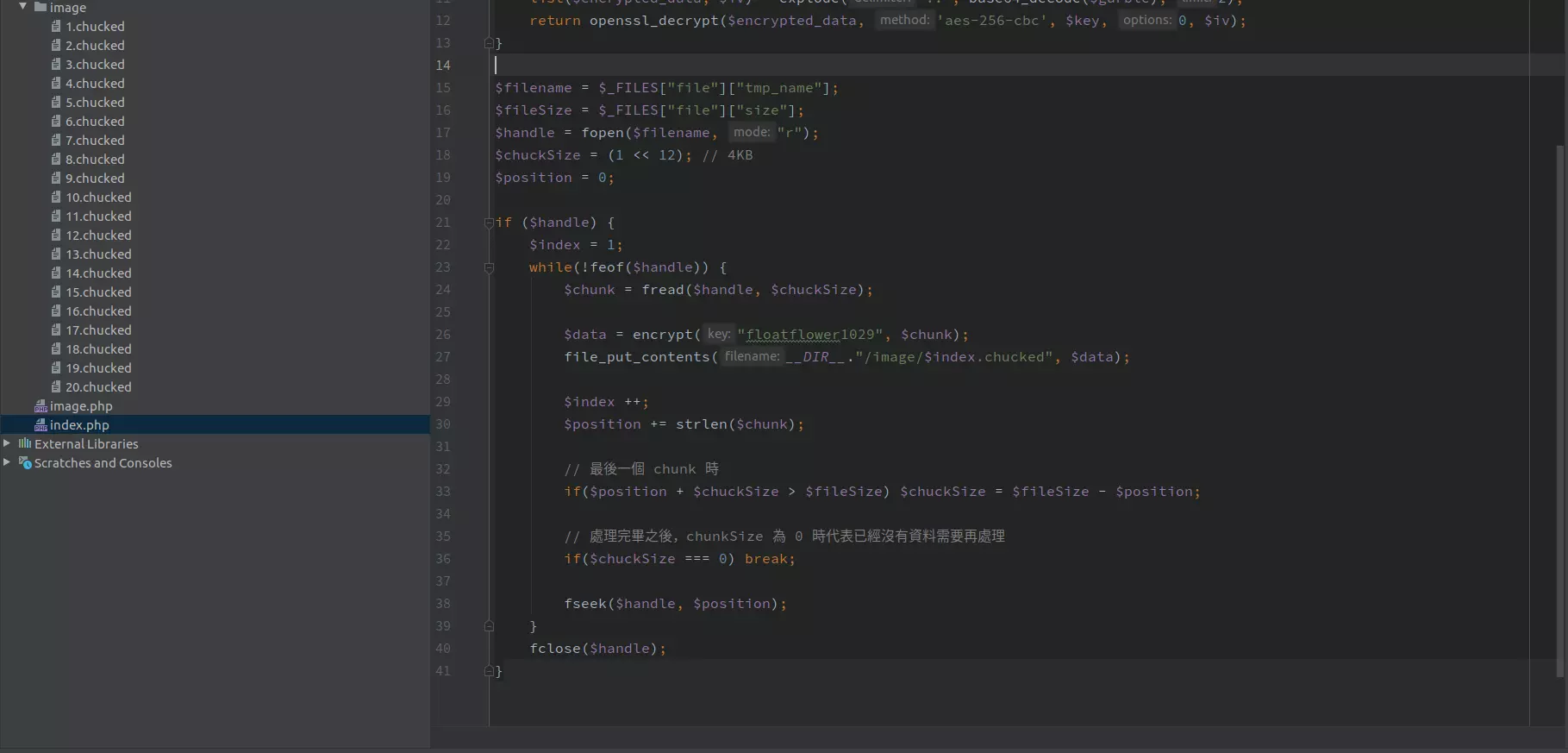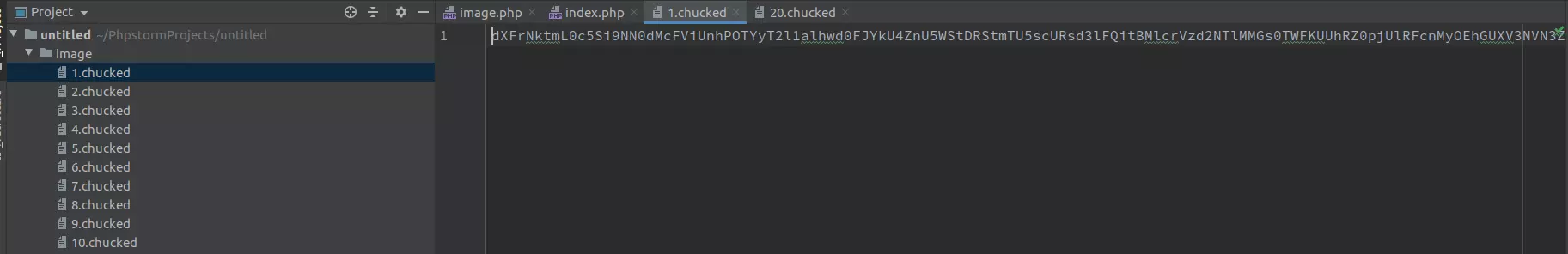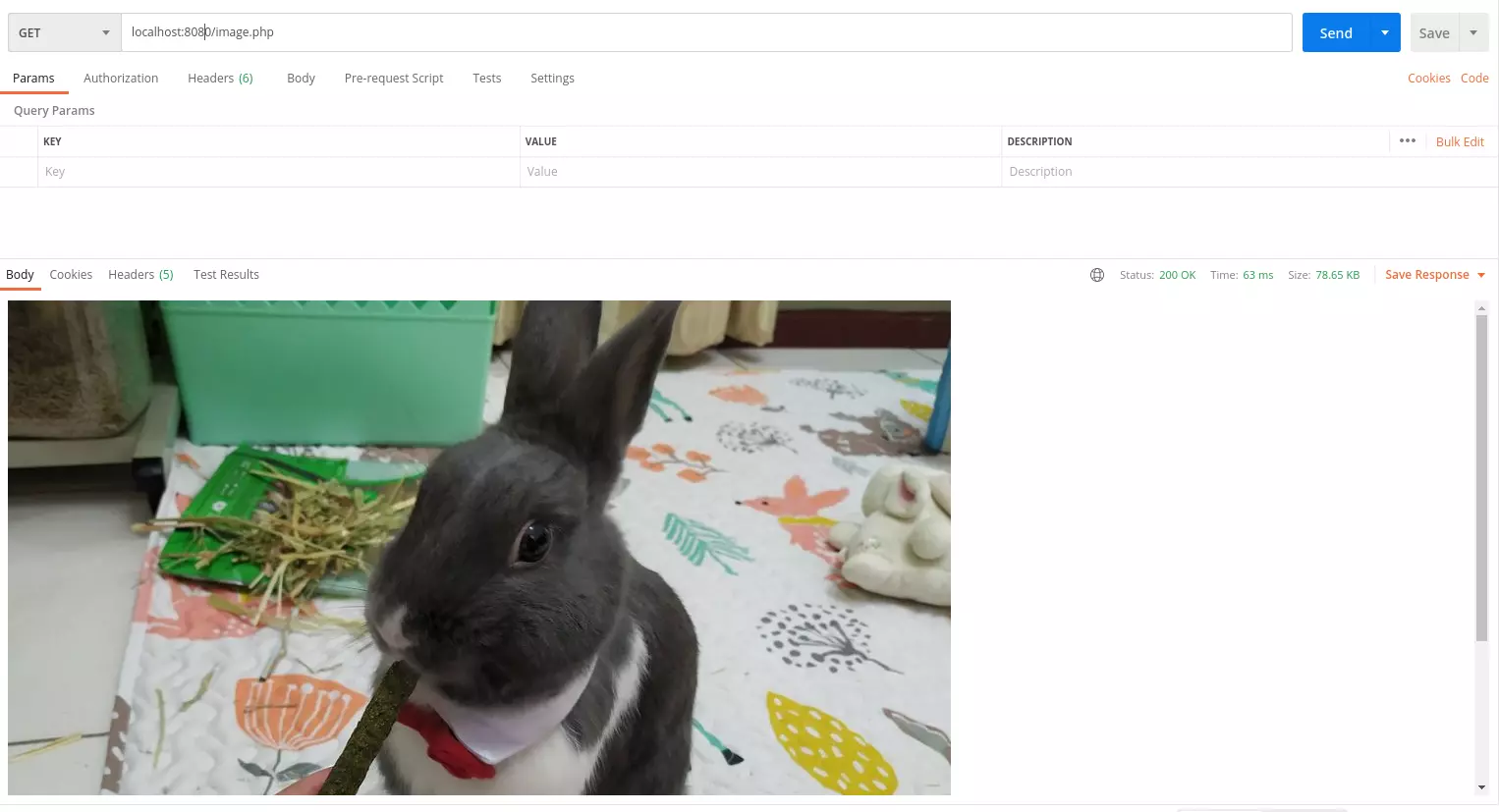### 背景

``````function encrypt(\$key, \$payload)
{
\$iv = openssl_random_pseudo_bytes(openssl_cipher_iv_length('aes-256-cbc'));
\$encrypted = openssl_encrypt(\$payload, 'aes-256-cbc', \$key, 0, \$iv);
return base64_encode(\$encrypted . '::' . \$iv);
}

``````

### 檔案分片並加密

``````<?php
// 加密函數
{
\$iv = openssl_random_pseudo_bytes(openssl_cipher_iv_length('aes-256-cbc'));
\$encrypted = openssl_encrypt(\$payload, 'aes-256-cbc', \$key, 0, \$iv);
return base64_encode(\$encrypted . '::' . \$iv);
}

// 解密函數
function decrypt(\$key, \$garble)
{
list(\$encrypted_data, \$iv) = explode('::', base64_decode(\$garble), 2);
return openssl_decrypt(\$encrypted_data, 'aes-256-cbc', \$key, 0, \$iv);
}

// 接受上傳並打開上傳後佔置於 /tmp 中的檔案
\$filename = \$_FILES["file"]["tmp_name"];
\$fileSize = \$_FILES["file"]["size"];
\$handle = fopen(\$filename, "r");
\$chuckSize = (1 << 12); // 4KB
\$position = 0;
\$index = 1;

if (\$handle) {
while(!feof(\$handle)) {

\$data = encrypt("floatflower1029", \$chunk);
file_put_contents(__DIR__."/image/\$index.chucked", \$data);

\$index ++;
\$position += strlen(\$chunk);

// 最後一個 chunk 時
if(\$position + \$chuckSize > \$fileSize) \$chuckSize = \$fileSize - \$position;

// 處理完畢之後，chunkSize 為 0 時代表已經沒有資料需要再處理
if(\$chuckSize === 0) break;

fseek(\$handle, \$position);
}
fclose(\$handle);
}
``````### 分片並傳送給用戶

``````<?php
// 加密函數
{
\$iv = openssl_random_pseudo_bytes(openssl_cipher_iv_length('aes-256-cbc'));
\$encrypted = openssl_encrypt(\$payload, 'aes-256-cbc', \$key, 0, \$iv);
return base64_encode(\$encrypted . '::' . \$iv);
}

// 解密函數
function decrypt(\$key, \$garble)
{
list(\$encrypted_data, \$iv) = explode('::', base64_decode(\$garble), 2);
return openssl_decrypt(\$encrypted_data, 'aes-256-cbc', \$key, 0, \$iv);
}

for(\$i = 1; \$i <= 20; \$i ++) {
// 解密並將檔案寫回客戶端
echo decrypt("floatflower1029", file_get_contents(__DIR__."/image/\$i.chucked"));
ob_flush();
flush(); // 強制輸出，不然一直 echo 不輸出再大檔案的時候還是會塞爆 Buffer。
}
``````